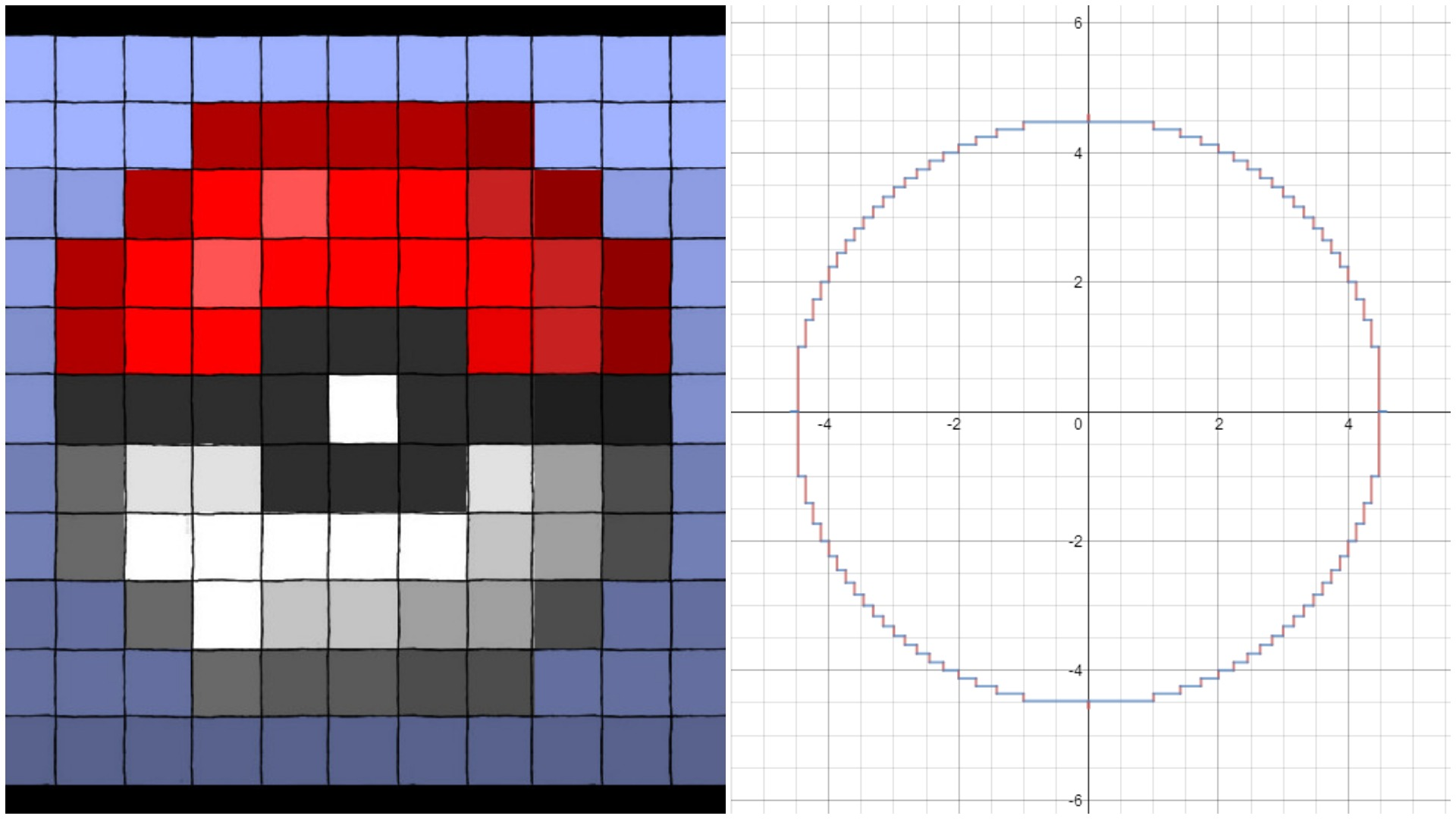Pixelated Poké Ball

Algebra Level 5${ x }^{ 2 }+\left\lfloor { y }^{ 2 } \right\rfloor =20\\ \left\lfloor { x }^{ 2 } \right\rfloor +{ y }^{ 2 }=20$

Find the area bounded by the two Cartesian equations above. If this area can be expressed in the form $\sum _{ n=1 }^{ Y }{ R\sqrt { n } } \left( \sqrt { G-n } -\sqrt { B-n } \right)$ with $R$, $G$, $B$, and $Y$ composite numbers, write your answer as $\dfrac { R\times G\times B }{ Y }$.

Bonus: Using this sum, generalize the bounded area with respect to $r$: ${ x }^{ 2 }+\left\lfloor { y }^{ 2 } \right\rfloor ={ r }^{ 2 }\\ \left\lfloor { x }^{ 2 } \right\rfloor +{ y }^{ 2 }={ r }^{ 2 }$

×